## Implementing Column Generation using SAS Optimization Decomposition to Solve Large-Scale Problems

Started ‎10-01-2019 by
Modified ‎10-01-2019 by
Views 3,760

The SAS Optimization decomposition algorithm (DECOMP) is a powerful variant of the column generation methods. The algorithm only requires the original (compact) formulation and the constraint partition. It does not require the explicit formulation of the subproblem nor the dual information.

In this article, I use the well-known Cutting Stock Problem to show how to use the DECOMP algorithm to solve its compact formulation. In my article Implementing Column Generation using SAS Optimization in SAS Viya, I also used the Cutting Stock Problem to show how in SAS Optimization one can formulate a typical Column Generation problem with clear iterations between Master and Subproblem. In this article, I compare both approaches: their mathematical models, their results, and when each of these approaches should be used.

SAS Optimization is the general-purpose optimization product that takes advantage of the distributed, cloud-based computational environment that SAS Viya provides. Large-scale problems benefit from running in a SAS Viya environment and their run time can be further shortened by parallelizing their subproblems. DECOMP can be implemented in distributed mode.

## DECOMP

DECOMP provides an alternative method of solving linear programs (LPs) and mixed integer linear programs (MILPs) by exploiting the ability to efficiently solve a relaxation of the original problem. In our experience, when the problem has a block-angular structure, or a great deal of symmetry, DECOMP often outperforms other algorithms. It automatically detects identical subproblems and uses Ryan-Foster branching if applicable. In Column Generation, one formulates the Master Problem and its subproblem.

The standard linear or mixed integer linear program has the formulation described by the first set of equations below. We can form a relaxation of the preceding mathematical program by removing the master constraints (matrices D and B). The resulting constraint system, defined by the matrix A and vector b, forms the subproblem. If the subproblem can be written in a block-angular form described by the second set of equations below, then DECOMP is often the best algorithm to use because it can achieve dramatic performance improvements over other branch-and-cut formulations.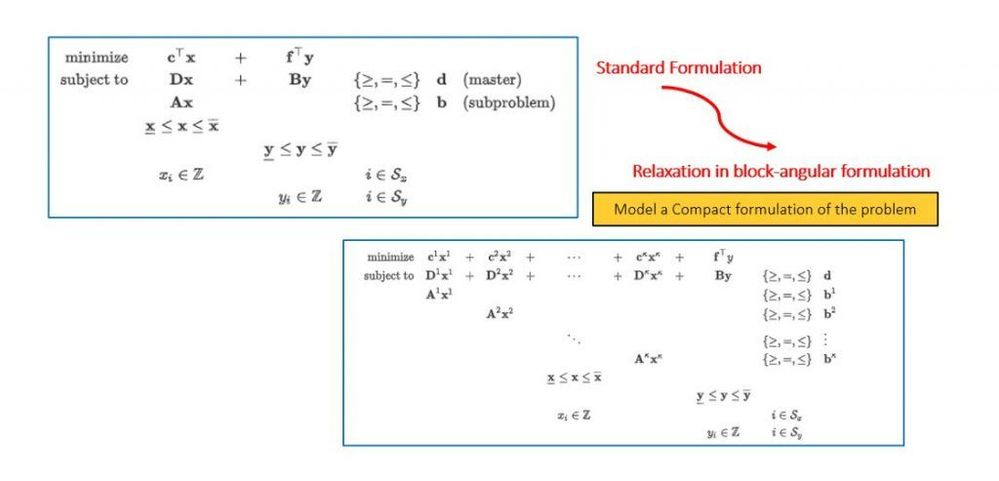Select any image to see a larger version.
Mobile users: To view the images, select the "Full" version at the bottom of the page.

The master problem is a linear program that is defined over a potentially large number of variables that represent the weights of a convex combination. The points in the convex combination satisfy the constraints that are defined in the subproblem. The master constraints of the original problem are enforced in this reformulated space. In this sense, DECOMP takes the intersection of two polyhedra: one defined by original master constraints and one defined by the subproblem constraints. Since the set of variables needed to define the intersection of the polyhedra can be large, the algorithm works on a restricted subset and generates only those variables (columns) that have good potential with respect to feasibility and optimality. This generation is done by using the dual information that is obtained by solving the master problem to price out new variables. These new variables are generated by solving the subproblems over the appropriate cost vector (the reduced cost in the original space). This generation is similar to the revised simplex method, except that the variable space is exponentially large and therefore is generated implicitly by solving an optimization problem. This idea of generating variables as needed is the reason why this method is often referred to as column generation.

DECOMP is used to solve MILPs, and other complex problems, such as Mixed Integer Nonlinear Programs (MINLP) or Network Problems, if they can be reformulated as MILPS. For examples and more information, view the SAS Optimization documentation for Mathematical Optimization Programming.

For an interesting application of the DECOMP algorithm solving a Network Problem read The Kidney Exchange Problem by Matthew Galati in Blogs on Operations Research with SAS.

## The Cutting Stock Problem using DECOMP Formulation

In Implementing Column Generation using SAS Optimization in SAS Viya, I described how a column generation heuristic works and how to implement it using SAS Optimization. Again, that heuristic can be used to solve complex problems, without the requirement of having a block- angular structure, or a great deal of symmetry. In that article, I used a trivial cutting stock example to illustrate the concepts, and the heuristics.

The image below shows the Column Generation formulation of the Cutting Stock Problem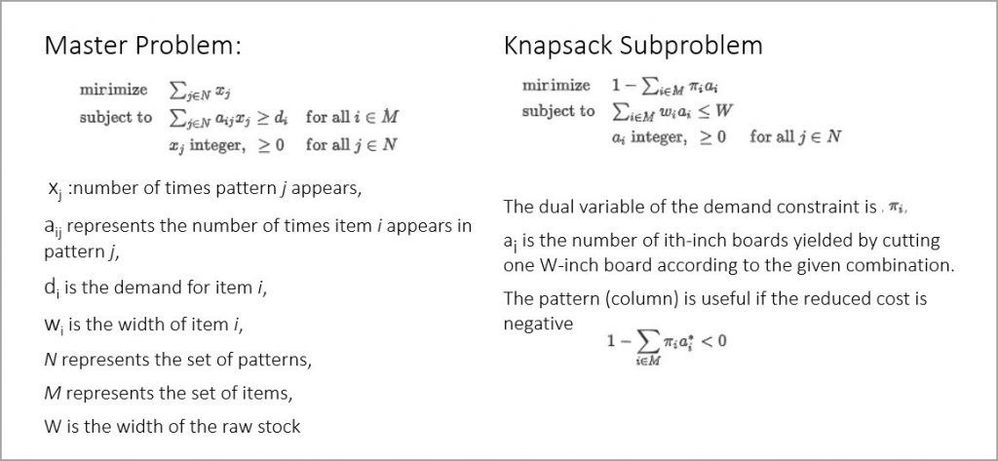In the DECOMP algorithm, one must write the compact formulation, and indicate where the partitioning is done by using the .block suffix on constraints (or use one of the built-in methods to automatically identify block structure). The blocks must be disjoint with respect to variables. Writing a clever mathematical formulation of the problem can be the difference between solving the problem, or not solving it at all.

One can formulate the Cutting Stock problem as a problem whose objective is to find the minimum number of raw-stock of fixed capacity (width) that are needed to satisfy the demand of items of varying size. The compact formulation of this problem is shown in the following image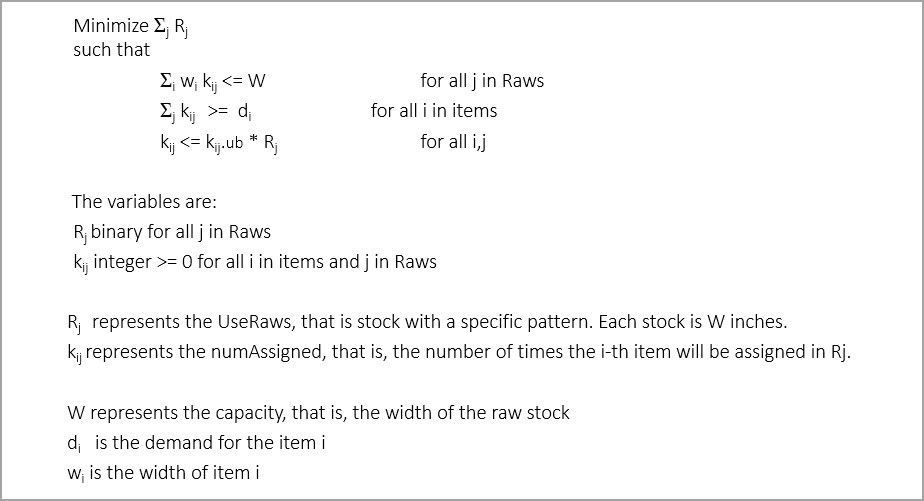The first constraint ensures that each pattern doesn't exceed the capacity constraint. The second constraint ensures that all items will have demand satisfied, and the third constraint effectively provides an upper bound for the numAssigned variable making the bounds tighter and the problem solves more efficiently. The partitioning is done on the first and the third constraints. In this example, DECOMP recognizes that Ryan-Foster branching can be used.

## Solution Code: The Cutting Stock Problem using DECOMP

The example I solved in my first article on Column Generation assumed that we want to cut a 91-inch log into smaller stocks of different widths and demands as shown in this tableHere is the code I wrote in SAS Studio V to implement the compact formulation of the Cutting Stock Problem using the DECOMP algorithm.

``````/* Cutting-stock problem solved using the Decomposition algorithm */
cas mySession sessopts=(caslib=CASUSER timeout=1800 locale="en_US");
libname CASUSER cas caslib=CASUSER ;

data CASUSER.indata;
input items demand width;
datalines;
1 78 25.5
2 40 22.5
3 30 20
4 30 15
;

%let capacity=91;

proc optmodel sessref="mySession";
/* declare parameters and sets */
num capacity = &capacity;
set ITEMS;
num demand {ITEMS};
num width {ITEMS};
num num_raws = sum {i in ITEMS} demand[i];
set RAWS = 1..num_raws;

read data CASUSER.indata into ITEMS= [items] demand width;

/* define problem */
var UseRaw {RAWS} binary;
var NumAssigned {i in ITEMS, j in RAWS} >= 0 <= capacity / width[i] integer;

minimize NumberOfRawsUsed = sum {j in RAWS} UseRaw[j];

/* respect capacity of each raw */
con knapsack_con {j in RAWS}:
sum {i in ITEMS} width[i] * NumAssigned[i,j] <= capacity
suffixes=(block=j);

/* satisfy demand of each item */
con demand_con {i in ITEMS}:
sum {j in RAWS} NumAssigned[i,j] >= demand[i];

/* if NumAssigned[i,j] > 0 then UseRaw[j] = 1 */
con ifthen_con {i in ITEMS, j in RAWS}:
NumAssigned[i,j] <= NumAssigned[i,j].ub * UseRaw[j]
suffixes=(block=j);

/* Decomposition: */
solve with milp / decomp varsel=ryanfoster;

/* These two lines will create the datasets that contain the optimal values for the decision variables */

create data CASUSER.CG_data from [i j] NumAssigned;
create data CASUSER.useRaw_data from [j] useRaw;

/* We want to extract the information on the patterns that are useful, that is, we want to know how to cut the raw stocks*/
create data CASUSER.CG_data_nonZero from [i j]={i in ITEMS, j in RAWS: NumAssigned[i,j]>0} patterns=NumAssigned;

create data CASUSER.useRaw_data_nonZero from [j]={j in RAWS: useRaw[j]>0} use=useRaw;

run;
quit;

/* After you have obtained the results, and you are done don't forget to terminate your CAS session */
/* CAS mySession TERMINATE; */
``````

## Analysis of Results

As expected, both formulations provide the same result and DECOMP is faster. There are several outputs from the code and one is the Solution Summary, shown below. Here you can see the results indicate the optimal solution uses 44 raw stocks.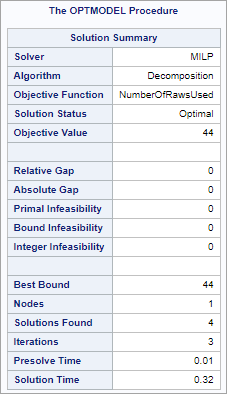Remember, the decision variables are:

• Rj represents the UseRaws, that is the raw stocks that are W-in wide which will have a specific pattern. For example, R44 represents the 44th raw stock.
• kij represents the number of times the i-th item will be assigned in Rj. For example, if k44,4 = 6, that means that the 4th item will be assigned 6 times to the raw stock number 44. The 4th item is 15 in long. Because 6 * 15 = 90, there will be one-inch waste out of the raw stock #44 because its length 91 is in.

There are two important output tables with the result from my code:

1. USERAW_DATA_NONZERO which contains UseRaws=1, the number of raw stocks, it has 44 rows
2. CG_DATA_ZERO which contains the k ij values that are non-zero; it has only 107 rows. I arranged these values into the 44 raw stock thus each row represents one raw stock, and the way it will be cut into the i-th different lengths. In a real-world application, the results are in the thousands or hundred-thousands rows, and they are passed into another custom application to further analyze and implement the results.

For the small example I used in this article, I used SAS Visual Analytics (VA) to visualize the results in CG_DATA_ZERO. Notice in the screenshot below that there are:

• 24 rows with pattern (2,1,0,1)
• 15 rows with pattern (2,0,2,0)
• 4 rows with pattern (0,4,0,0)
• 1 row with pattern (0,0,0,6).

Similarly, for the other rows. Again, these results are the same as the ones obtained in the first article.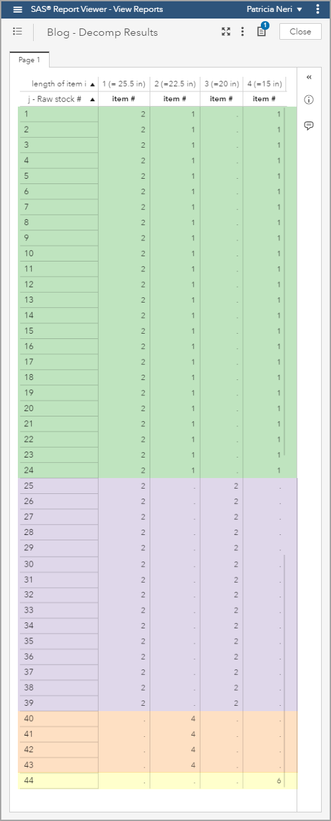The 24 rows with pattern (2,1,0,1) indicate that 24 91-in raw stocks will be cut as two 25.5", one 22.5" and one 15" logs. Similarly, for the other rows. Again, these results are the same as the ones obtained in the first article.

## Using Open Source to execute SAS Optimization’s DECOMP

The sasoptpy modeling package gives you the ability to write optimization models in Python and solve them using SAS Optimization solvers. The sasoptpy package provides a convenient modeling framework for programmers who already use Python. This package is available on GitHub, as there are other SAS open source projects.

The SAS Global Forum paper, Optimization Modeling with Python and SAS Viya, provides examples on how to use sasoptpy, and one of them is the Kidney Exchange Problem. This paper provides a clear definition of the problem, data generation, how to define the Mathematical model, call DECOMP, and use Matplotlib and NetworkX to graph the results.

## Summary

Column Generation Techniques are alive and well, they solve a variety of large-scale complex and useful problems. Column Generation can be easily implemented using SAS Optimization and take advantage of distributed environments. If the problem has a block-angular structure, or a great deal of symmetry, our recommendation is to try DECOMP.

## References

Galati, M.V. (2009). “Decomposition in Integer Linear Programming.” Ph.D. diss., Lehigh University.

## Acknowledgement

To Rob Pratt for sharing his knowledge on Mathematical Optimization and for providing feedback on the first draft of this article.

Version history
Last update:
‎10-01-2019 12:09 PM
Updated by:Contributors
Article Labels
Article Tags